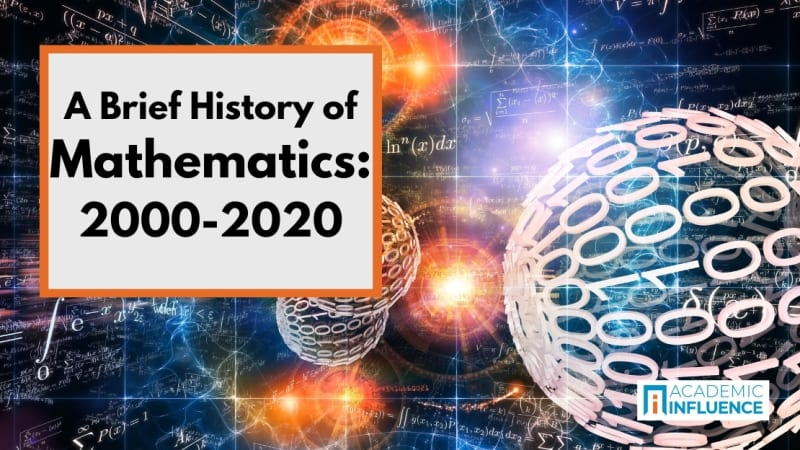# A Brief History of Mathematics: 2000-2020We explore the field of mathematics from 2000-2020.

By the turn of the century, computer science was well established as an important and vital tool for exploring mathematics as well as proving previously stubborn theorems and conjectures. Curiously, mathematics in the 21st century has both demonstrated the dominance of computation and continued to highlight the importance of pure mathematics as performed by human mathematicians. Let’s have a closer look.

In 2002, a number of mathematicians, notably Manindra Agrawal , Nitin Saxena , and Neeraj Kayal developed a powerful procedure for determining whether a given number is prime (divisible only by 1 and itself). The procedure or algorithm, known as the AKS primality test, is “polynomial,” which means that it can be computed in a reasonable amount of time, as well as general, which means that given any integer as input, the output of the AKS test determine whether the input is prime or not. This is a remarkable result in mathematics, but in fact it was developed as an algorithm in what we typically consider computer science research. This trend of incubating new mathematical innovations within the field of computer science continues to the present day.

On the other hand, some startling and important results have appeared in the 21st century that are examples of pure mathematics, rather than computer methods. Importantly, Grigori Perelman proved famous mathematician Henri Poincaré ’s 1904 conjecture about the ”3-sphere,” a result in topology, using fundamental mathematical concepts and tools in topology and geometry. Perelman showed that math was still alive and kicking in the 21st century, a century that also witnessed supercomputer clusters applied to computing the ratio pi out to trillions of digits—a result which would have been impossible without supercomputers. Yet Perelman also used pure mathematics to prove a theorem in geometry (Thurston’s geometrization conjecture) in addition to the shocking 3-sphere proof, both in 2003. Not only did the Russian mathematician offer the proofs, but he turned down the Fields Medal as well as a one million dollar prize, remarking that finding the proof was enough. This is as pure as mathematics get!.

And in 2002, the Romanian mathematician Preda Mihăilescu proved true the 158 year old Catalan’s conjecture, a long-unsolved puzzle in number theory. And Ben Green and Terence Tao proved another central problem in number theory, now known as the Green-Tao Theorem. In 2009 the “fundamental lemma” (also known as Langland’s program after mathematician Robert Langlands , who first formulated the problem) was proved by Ngô Bảo Châu . In 2013, Yitang Zhang proved an important property about primes (the first finite bound on gaps between prime numbers), and in 2014 an amazing and longstanding problem known as Kepler’s Conjecture, after the 17th century mathematician and astronomer Johannes Kepler , was solved. Thus the current century has proved that pure mathematics has not been replaced by computer science, even as important results (especially in factoring, primes, and large numbers) have been attacked using computing. It is indeed an interesting time for mathematics.

A modern history of mathematics would not be complete without noting an important trend started in the 20th century and continued into the present-day—large scale collaboration. In 2004, the classification of finite simple groups was completed, after fifty years of work in collaboration with a large team of mathematicians and researchers. Indeed, as modern technology has enabled and facilitated effective large scale collaboration, we might anticipate such efforts on future problems to yield similarly impressive results.

***

Find out which influencers have most contributed to advancing the field of mathematics over the last two decades with a look at The Most Influential People in Mathematics, for the years 2000 – 2020.

To find out which schools are driving the mathematics field forward today, check out The Most Influential Schools in Mathematics, for the years 2000 – 2020.

Get 77 helpful free math resources online, with an emphasis on BIPOC student needs.

Or, look back in time for A Brief History of Mathematics: 1950-2000.